Courses

# Time Domain Analysis of Control Systems - 2

## 20 Questions MCQ Test Topicwise Question Bank for Electrical Engineering | Time Domain Analysis of Control Systems - 2

Description
This mock test of Time Domain Analysis of Control Systems - 2 for Electrical Engineering (EE) helps you for every Electrical Engineering (EE) entrance exam. This contains 20 Multiple Choice Questions for Electrical Engineering (EE) Time Domain Analysis of Control Systems - 2 (mcq) to study with solutions a complete question bank. The solved questions answers in this Time Domain Analysis of Control Systems - 2 quiz give you a good mix of easy questions and tough questions. Electrical Engineering (EE) students definitely take this Time Domain Analysis of Control Systems - 2 exercise for a better result in the exam. You can find other Time Domain Analysis of Control Systems - 2 extra questions, long questions & short questions for Electrical Engineering (EE) on EduRev as well by searching above.
QUESTION: 1

### The system shown in figure below has unit step input.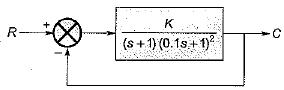In order that the steady state error is 0.1, the value of K required should be

Solution: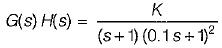Since Input is unit step, therefore steady state error is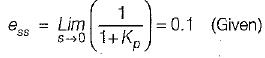Here,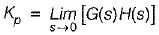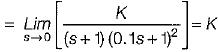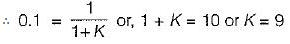QUESTION: 2

### The steady-state error coefficients for a system are Kp = ∞ , Kv = finite constant and Ka = 0. The type of the system is

Solution:

We have,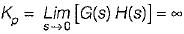= finite constant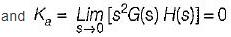From above values of Kp, Kv and Ka we conclude that the type of the system should be one.

QUESTION: 3

### The steady-state error of a feedback control system with an acceleration input becomes finite in a

Solution:

Steady state error with an acceleration input having an amplitude of A is given by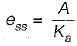where,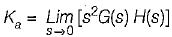Hence, if the type of the system = 2, then Ka = some non-zero value or finite value due to which we will get some finite vaiue of Ka.

QUESTION: 4

Consider the unity feedback system shown below:​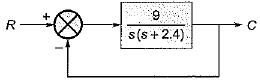The settling time of the resulting second order system for 2% tolerance band will be

Solution:

The characteristic equation of the given closed loop system is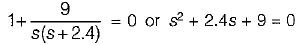Comparing above equation with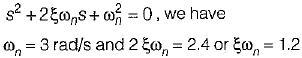Thus, setting time for 2% tolerance band is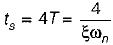or,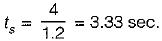QUESTION: 5

For a control system, the Laplace transform of error signal e(t) is given by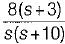. The steady state value of the error will be

Solution:

Given,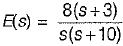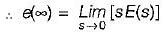(Using final value theorem)
or,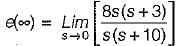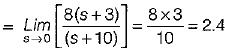QUESTION: 6

For a type one system, the steady-state error due to step input is equal to

Solution: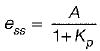where,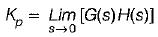(Here, A = magnitude of step input)
Since system is type -1, therefore Kp = ∞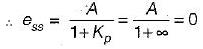QUESTION: 7

For an unity feedback control system with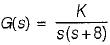the value of K for damping ratio of 0.5 is

Solution:

Characteristic equation is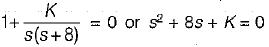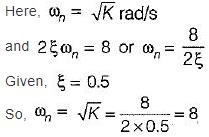or, K = 64

QUESTION: 8

The damping ratio of a system having the characteristic equation s2 + 2s + 8 = 0 is

Solution:

Given, s2 + 2s + 8 = 0
Here, ωn = √8 rad/s = 2√2 rad/sec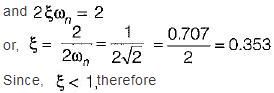system is underdamped.

QUESTION: 9

The closed-loop transfer function of a unity - feedback system is given by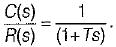The steady state error to a unit ramp input is:

Solution:

Given,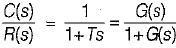(Since H(s) = 1)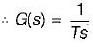For a unit ramp input,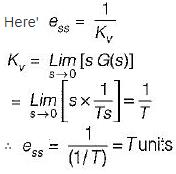QUESTION: 10

The peak overshoot of step-input response of an underdamped second-order system is an indication of

Solution:

If damping of system increases, peak overshoot Mp decreases and vice-versa.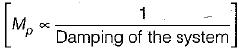QUESTION: 11

Consider the position control system shown below: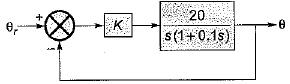The value of K such that the steady state error is 20° for input θr = 300t rad/sec, is

Solution:

Input = 300t rad/sec = ramp input of magnitude A = 300.
For ramp input, steady state error is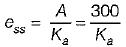where,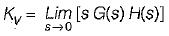Now, from given block diagram, we have: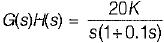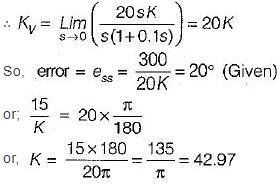So, value of gain is K = 42.97

QUESTION: 12

Assertion (A): With the increase in bandwidth of the system the response of the system becomes fast.
Reason (R): Damping ratio of the system decreases with the increase in bandwidth.

Solution:

When BW is increased, the system response becomes fast due to fait in rise time (tr).
With the increase in bandwidth of the system, damping ratio (ξ) decreases.
Thus, both assertion and reason are true but, reason is not the correct explanation of assertion.

QUESTION: 13

For the stable system described by the block diagram shown below, Match List - I (Static error coefficients) with List - II (Values) and select the correct answer using the codes given below the lists: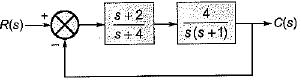List-I
A. Ka
B. Kv
C. Kp

List-II
1. ∞
2. 0
3. 2
4. -1

Codes: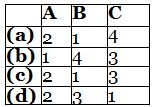Solution:

We have,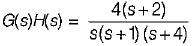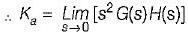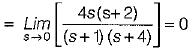Also,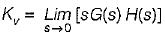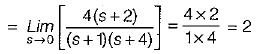and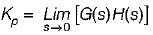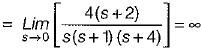QUESTION: 14

The unit step response of a system is given by c(t) - 1 + 0.25 e-50t - 1.25 e-10t
The given system is

Solution:

Given,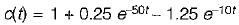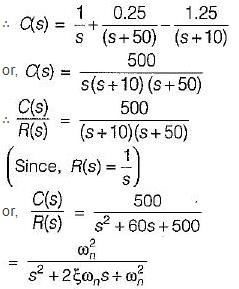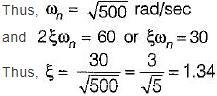Since, ξ > 1, therefore given system is overdamped.

QUESTION: 15

Match List - I (Transfer function of systems) with List - II (Nature of damping) and select the correct answer using the codes given below the lists: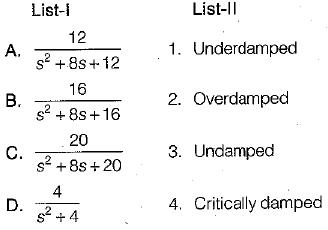Codes: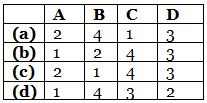Solution:

Characteristic equations are:
• s2 + 8s + 12 = 0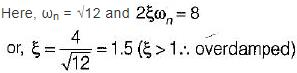• s2 + 8s + 16 = 0
Here, ωn = 4 and 2 ξωn = 8
or, ξ = 1 (critically damped)
• s2 + 8s + 20 = 0
Here, ω = √20
and 2ξω= 8
or, ξ = 0.894 (ξ < 1 ∴ underdamped)
• s2 + 4 = 0
or, ωn = 2
and ξ = 0 (∴ undamped)

QUESTION: 16

The block diagram of an electronic pacemaker is given in figure below.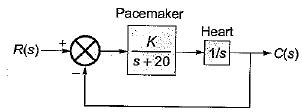What is the value of K for which the steady-state error to a unit ramp input is 0.02?

Solution:

Here,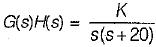As the input is a unit ramp function, therefore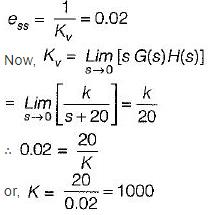QUESTION: 17

Which one of the following equations gives the steady-state error for a unity feedback system excited by r(t) = 2 + 5t + 2t2 ?

Solution:

Given, r(t) = 2 + 5t + 2t2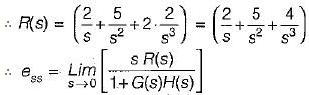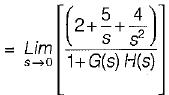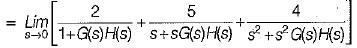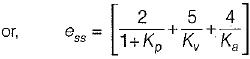QUESTION: 18

An unity feedback control system with closed loon transfer function is given by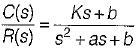The steady state error due a unit ramp input response is

Solution: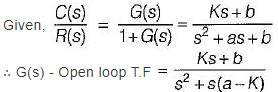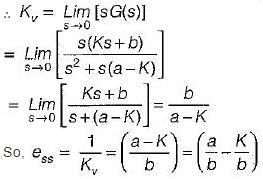QUESTION: 19

The second order approximation using dominant pole concept for the transfer function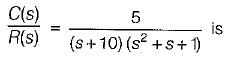Solution:

Given,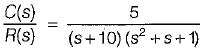In time constant form,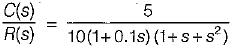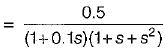Using dominant pole concept, the given transfer function can be approximated to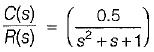QUESTION: 20

The unit step response of the system represented by the block diagram shown below is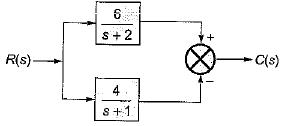Solution:

From given block diagram, we have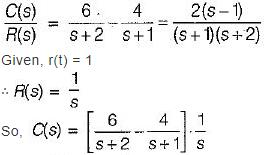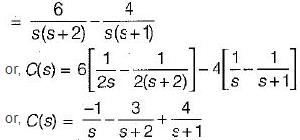∴ c(t) = (4e-t - 3e-2t - 1)
= Required step response